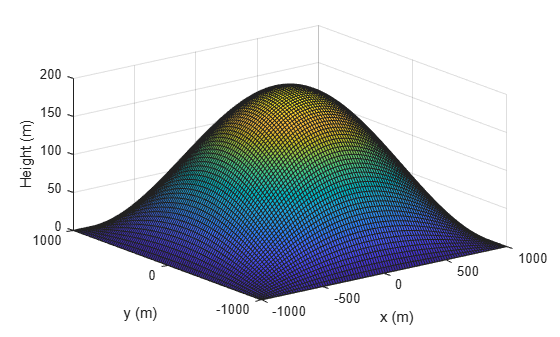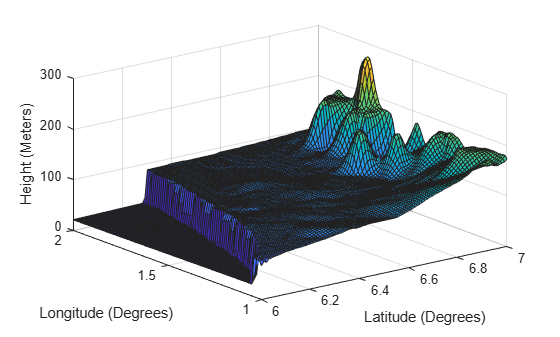# GroundSurface

Ground surface belonging to tracking scenario

Since R2022a

## Description

The `GroundSurface` object defines a ground surface object belonging to a `trackingScenario` object. You can use the `GroundSurface` object to model terrain in a scenario and query the occlusion status of line-of-sight between two points in the scenario.

## Creation

Create `GroundSurface` objects using the `groundSurface` object function of the `trackingScenario` object.

## Properties

expand all

Terrain data for the surface, specified as an M-by-N real-valued matrix, or a string scalar specifying a Digital Terrain Elevation Data (DTED) file name.

• M-by-N real-valued matrix — The matrix values represent the height data of an area defined by the `Boundary` property of the ground surface object. The object extends the height data in the matrix to the area. The object automatically fills heights of unspecified points using linear interpolation. M and N must both be greater than 3.

• String scalar or character vector specifying DTED file name — To use this option, you must specify the `IsEarthCentered` property of the tracking scenario as `true`. In this case, the object specifies the terrain heights for the area using those defined in the DTED file. The ground surface object automatically fills unspecified data in the DTED file using linear interpolation. If you want to use only a part of the terrain defined in the DTED file, specify the `Boundary` property as your desired subset of the terrain area defined in the DTED file. Otherwise, specify the `Boundary` property as the whole area defined in the DTED file.

Data Types: `single` | `double` | `char`

Boundary of the surface, specified as a 2-by-2 matrix of real values with the form `[xmin xmax; ymin ymax]`. When the `IsEarthCentered` property of tracking scenario object is specified as:

• `false` — Specify `xmin`, `xmax`, `ymin` `ymax`, in meters, as Cartesian coordinates in the reference frame of the scenario, where `xmin` < `xmax`, and `ymin` < `ymax`

• `true` — Specify `xmin` and `xmax` as the minimum and maximum latitudes of the geodetic frame in degrees, where `xmin` < `xmax`. Specify `ymin` and `ymax` as the minimum and maximum longitudes of the geodetic frame in degrees. If `ymax` < `ymin`, the object wraps `ymax` to `ymax` + `360`.

Data Types: `single` | `double`

Reference height of the terrain data, specified as a scalar in meters. Specify the terrain data relative to this reference height.

Data Types: `single` | `double`

## Object Functions

 `height` Height of surface in tracking scenario `occlusion` Determine occlusion status by surface

## Examples

collapse all

Create a mesh grid that spans from -1000 meters to 1000 meters in both the x- and y-directions.

`[x,y] = meshgrid(linspace(-1000,1000,500));`

Specify the height for each mesh grid point.

`z = 200*cos(x*pi/2000).*cos(y*pi/2000);`

Create a tracking scenario and add a ground surface object to the tracking scenario. Specify the boundary of the surface area.

```scene = trackingScenario; surface = groundSurface(scene,Terrain=z,Boundary=[-1e3 1e3; -1e3 1e3])```
```surface = GroundSurface with properties: Terrain: [500x500 double] ReferenceHeight: 0 Boundary: [2x2 double] ```

Note that the `SurfaceManager` property of the tracking scenario now contains the created `GroundSurface` object.

`manager = scene.SurfaceManager`
```manager = SurfaceManager with properties: UseOcclusion: 1 Surfaces: [1x1 fusion.scenario.GroundSurface] ```
`manager.Surfaces`
```ans = GroundSurface with properties: Terrain: [500x500 double] ReferenceHeight: 0 Boundary: [2x2 double] ```

Visualize the surface using the `helperGetTerrainMap` helper function, attached to this example.

```xSamples = linspace(-1e3,1e3,100); ySamples = linspace(-1e3,1e3,100); helperGetTerrainMap(surface,xSamples,ySamples); xlabel("x (m)") ylabel("y (m)") zlabel("Height (m)")```Create a tracking scenario and specify its `IsEarthCentered` property as `true`.

`scene = trackingScenario(IsEarthCentered=true);`

Add a ground surface based on a DTED file, covering from 6 to 7 degrees in latitude and from 1 to 2 degrees in longitude.

```terrain = "n06.dt0"; boundary = [6 7; % Latitude in degrees 1 2]; % Longitude in degrees surface = groundSurface(scene,Terrain=terrain,Boundary=boundary);```

Sample the area using a 100-by-100 grid map.

```samples = 100; latitudes = linspace(6,7,samples); longitudes = linspace(1,2,samples); positions = [latitudes; longitudes];```

Plot the terrain using the `helperGetTerrrainMap` helper function, attached to this example`.`

```helperGetTerrainMap(surface,latitudes,longitudes); xlabel("Latitude (Degrees)"); ylabel("Longitude (Degrees)"); zlabel("Height (Meters)");```Create a tracking scenario object.

`scene = trackingScenario;`

Specify the terrain as `magic(4)` and define the boundary of the terrain as a square.

`terrain = magic(4)`
```terrain = 4×4 16 2 3 13 5 11 10 8 9 7 6 12 4 14 15 1 ```
`boundary = [0 100; 0 100];`

Create the ground surface and add it to the scenario.

`surface = groundSurface(scene,Terrain=terrain,Boundary=boundary)`
```surface = GroundSurface with properties: Terrain: [4x4 double] ReferenceHeight: 0 Boundary: [2x2 double] ```

Get the heights of the surface at the center and its four corners.

`h0 = height(surface,[50 50]')`
```h0 = 8.5000 ```
`h1 = height(surface,[0 0]')`
```h1 = 16 ```
`h2 = height(surface,[100 0]')`
```h2 = 13 ```
`h3 = height(surface,[0 100]')`
```h3 = 4 ```
`h4 = height(surface,[100 100]')`
```h4 = 1 ```

Create a tracking scenario object.

`scene = trackingScenario;`

Specify the terrain and define the boundary of the terrain as a square centered at the origin.

```terrrain =[0 10 0; 0 10 0; 0 10 0]; boundary = [-100 100; -100 100];```

Create the ground surface and add it to the scenario.

`surface = groundSurface(scene,Terrain=terrrain,Boundary=boundary)`
```surface = GroundSurface with properties: Terrain: [3x3 double] ReferenceHeight: 0 Boundary: [2x2 double] ```

Query the occlusion status of the line-of-sight vector between the corner point (–100, –100, 0) and the other corner point (100, 100, 20). The ground surface occludes the line-of-sight.

`occlusion(surface,[-100 -100 0],[100 100 20])`
```ans = logical 1 ```

Raise the height of the first corner point. Now the ground surface no longer occludes the line-of-sight.

`occlusion(surface,[-100 -100 10],[100 100 20])`
```ans = logical 0 ```

## Version History

Introduced in R2022a